Latest Banking jobs   »   Reasoning Ability, Inequalities Quiz For SBI...

# Reasoning Ability, Inequalities Quiz For SBI PO, Clerk Prelims 2021- 8th July

Directions (1-5): Study the information carefully and answer the question given below.
Eight persons are living on an eight-floor building, ground floor is numbered 1 and topmost floor is numbered as 8. D lives on an even number floor below 5th floor. There is only one person lives between D and C. G lives on one of the even number floors but not on the top floor. There are two persons living between C and A who lives below E. H lives on a floor immediate above the B’s floor. The total number of persons living between F and B is same as the total number of persons living between F and E.

Q1. Who among the following lives on third floor?
(a) H
(b) G
(c) F
(d) E
(e) None of these

Q2. How many persons live between H and D?
(a) Two
(b) More than Three
(c) Three
(d) One
(e) None

Q3. G lives on which of the following floor?
(a) 6th floor
(b) 4th floor
(c) 2nd floor
(d) 8th floor
(e) None of these

Q4. Who among the following lives immediate below D?
(a) G
(b) F
(c) A
(d) B
(e) None of these

Q5. Which of the following statement is true about F?
(a) E lives one of the floor above F’s floor
(b) Only two floors between F and D
(c) F lives immediate above G
(d) F lives on an even numbered floor
(e) None of these

Directions (6-8): Study the following information carefully and answer the given questions.
Point S is 40 m towards the North of Point P. Point Q is 15 m towards the West of point S. Point U is 25 m towards the West of point S. Point T is 20 m towards the South of point Q. Point R is 15 m towards the East of point T. Point V is 15 m towards the West of point P.

Q6. Which of the following points are in a straight line?
(a) S, T, P
(b) T, V, R
(c) S, Q, V
(d) T, V, Q
(e) U, Q, R

Q7. P is in which direction with respect to R?
(a) South
(b) North
(c) East
(d) West
(e) Cannot be determined

Q8. If a person walks 10 m towards East from point U and then takes a right turn, which of the following points would he reach first?
(a) V
(b) S
(c) T
(d) P
(e) R

Directions (9-11): Study the following information carefully and answer the questions given below.
There are some persons sitting in a row facing in North direction. Two persons sit between C and E. Four persons sit between C and D. B is a neighbour of D. Only five persons sit to the right of C. As many persons sit between C and E as sit between B and E. A sits third from one of extreme ends of the row and is not neighbour of D. Only three persons sit to the left of E.
Q9. If E sits between D and F, then what is the position of F with respect to A?
(a) Fifth to the right
(b) Seventh to the right
(c) Fifth to the left
(d) Seventh to the left
(e) None of these

Q10. How many persons can sit in a row?
(a) Twelve
(b) Ten
(c) Fifteen
(d) Eleven
(e) None of these

Q11. What is the position of E with respect to D?
(a) Fifth to right
(b) Second to the right
(c) Fifth to the left
(d) Seventh to the left
(e) None of these

Q12. How many pairs of letters are there in the word “SECURITY”, which have as many letters between them in the word as in alphabetical series?
(a) None
(b) One
(c) Two
(d) Three
(e) Four

Direction (13-15): In this question, relationship between different elements is shown in the statements. The statements are followed by conclusions. Study the conclusions based on the given statement and select the appropriate answer.
Q13. Statements: A≥B=Q≤P<J≤Y; Z≥A>X; A<M
Conclusions: I.B<Y II.X≥J
(a) Only conclusion I is true.
(b) Neither conclusion I nor II is true.
(c) Only conclusion II is true.
(d) Both conclusions I and conclusion II are true.
(e) Either conclusion I or II is true.

Q14. Statements: M>A≥B=Q;Z≥A>X; Q≤P<J≤Y
Conclusions: I.Z=Q II.Z>Q
(a) Only conclusion I is true.
(b) Neither conclusion I nor II is true.
(c) Only conclusion II is true.
(d) Both conclusion I and conclusion II are true.
(e) Either conclusion I or II is true.

Q15. Statements: T<R; X<G<R=A≤S
Conclusions: I.G<S II.S>T
(a) Only conclusion I is true.
(b) Neither conclusion I nor II is true.
(c) Only conclusion II is true.
(d) Both conclusions I and conclusion II are true.
(e) Either conclusion I or II is true.

Practice More Questions of Reasoning for Competitive Exams:

###### SBI Clerk Study Plan 2021

Solutions

Solutions (1-5):
Sol.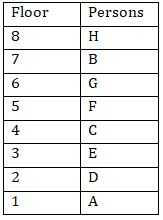S1. Ans.(d)
S2. Ans.(b)
S3. Ans.(a)
S4. Ans.(c)
S5. Ans.(b)

Solution (6-8):
Sol.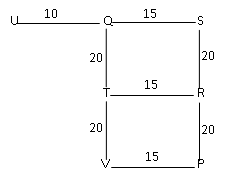S6. Ans.(d)
S7. Ans.(a)
S8. Ans.(c)

Solutions (9-11):
Sol.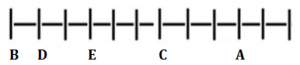S9. Ans.(e)
S10. Ans.(a)
S11. Ans.(b)

S12. Ans.(d)
Sol.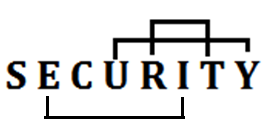S13.Ans.(a)
Sol. I.B<Y (TRUE) II.X≥J (FALSE)

S14.Ans.(e)
Sol. I.Z=Q (FALSE) II.Z>Q (FALSE)

S15.Ans.(d)
Sol. I.G<S (TRUE) II.S>T (TRUE)#### Congratulations!Incorrect details? Fill the form again here

•Important Current Affairs Quiz for Bank ...
•Important Current Affairs Quiz for Bank ...
•Reasoning Ability Quiz For IBPS RRB PO C...
•Reasoning Ability Quiz For IBPS Clerk Pr...
•Reasoning Ability Quiz For IBPS RRB PO P...
•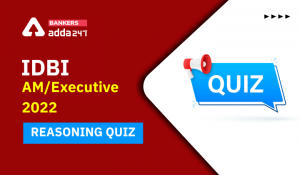Reasoning Ability Quiz For IDBI AM/Execu...